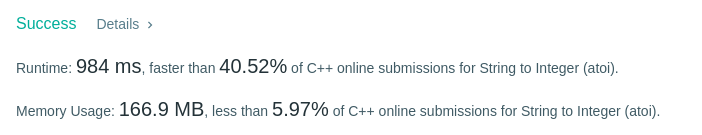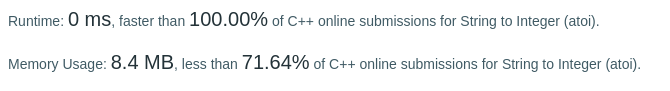# Coding Spirit

0%

Algorithm Puzzles everyday every week sometimes: String to Integer (atoi)

## Puzzle

Puzzle from leetcode:

Implement atoi which converts a string to an integer.

The function first discards as many whitespace characters as necessary until the first non-whitespace character is found. Then, starting from this character, takes an optional initial plus or minus sign followed by as many numerical digits as possible, and interprets them as a numerical value.

The string can contain additional characters after those that form the integral number, which are ignored and have no effect on the behavior of this function.

If the first sequence of non-whitespace characters in str is not a valid integral number, or if no such sequence exists because either str is empty or it contains only whitespace characters, no conversion is performed.

If no valid conversion could be performed, a zero value is returned.

Note:

Only the space character ‘ ‘ is considered as whitespace character.
Assume we are dealing with an environment which could only store integers within the 32-bit signed integer range: [−231, 231 − 1]. If the numerical value is out of the range of representable values, INT_MAX (231 − 1) or INT_MIN (−231) is returned.
Example 1:

Example 2:

Example 3:

Example 4:

Example 5:

## Solution

My first solution is using regex to filtrate incorrect inputs and find out the numerical:

Then :I realized that maybe using regex is not a good idea since there is no need to traversal string more than once… After some modification, here is my solution 2:

Result I got:0 ms and better than 100%…